#### Handling obstacles

A simple way to handle obstacles is to determine for each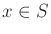whether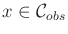. This can be computed and stored in an array before the value iterations are performed. For rigid robots, this can be efficiently computed using fast Fourier transforms . For each,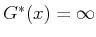. No value iterations are performed on these states; their values must remain at infinity. During the evaluation of (8.59) (or a higher dimensional version), different actions are attempted. For each action, it is required that all of the interpolation neighbors of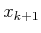lie in. If one of them lies in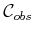, then that action produces infinite cost. This has the effect of automatically reducing the interpolation region,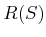, to all cubes whose vertices all lie in, as shown in Figure 8.21b. All samples inare assumed to be deleted from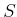in the remainder of this section; however, the full grid is still used for interpolation so that infinite values represent the obstacle region.

Note that as expressed so far, it is possible that points inmay lie inbecause collision detection is performed only on the samples. In practice, either the grid resolution must be made fine enough to minimize the chance of this error occurring or distance information from a collision detection algorithm must be used to infer that a sufficiently large ball around each sample is collision free. If an interpolation region cannot be assured to lie in, then the resolution may have to be increased, at least locally.

Steven M LaValle 2020-08-14